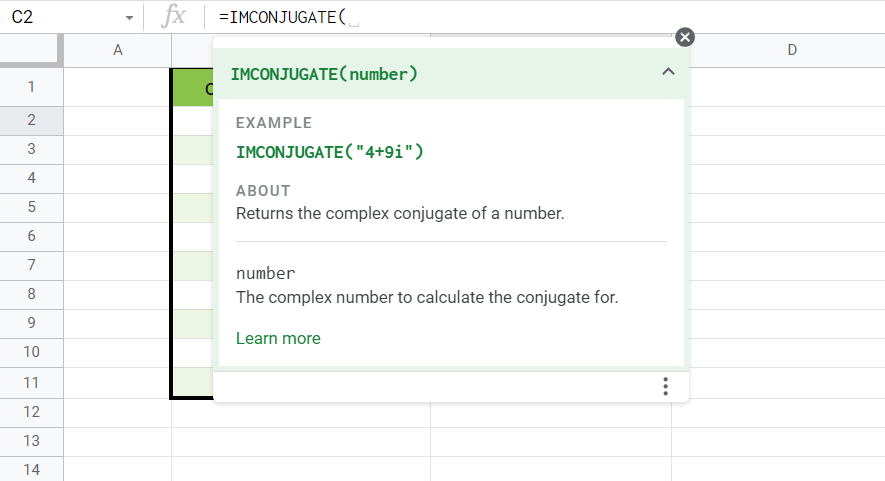# How To Use IMCONJUGATE Function in Google Sheets

The IMCONJUGATE function in Google Sheets is useful when you want to find the complex conjugate of a given complex number.

The complex conjugate of a number has an equal real coefficient and an imaginary coefficient equal in magnitude but opposite in sign. For example, the complex number in the form a+bi would have a conjugate of a-bi.

The rules for using the `IMCONJUGATE` function in Google Sheets are as follows:

• The function simply requires a complex number in the a+bi or a+bj format.
• The function returns the complex conjugate of the given complex number.

Let’s take a look at a quick example!

In this example, we have a table of complex numbers that we would like to plot in a 2D plane. Additionally, we would like to plot the complex conjugate of each given complex number.

In mathematics, the real coefficient corresponds to a position in the x-axis, and the imaginary coefficient represents the y-axis. Geometrically, the conjugate is the reflection of a given point over the real axis (x-axis). This relationship can be seen in the diagram below.Performing these computations is difficult at first glance since complex numbers are usually treated as text by Google Sheets. Luckily, we have several functions that deal specifically with operations on complex numbers. In this case, we can simply use the `IMCONJUGATE` function to retrieve the complex conjugate of each given complex number. Later, we’ll go step-by-step into how we can accomplish this.

For now, let’s learn how to write the `IMCONJUGATE` function ourselves in Google Sheets and later test out the function with a sample dataset.

## The Anatomy of the IMCONJUGATE Function

So the syntax (the way we write) the `IMCONJUGATE` function is as follows:

``` =IMCONJUGATE(number)
```

Let’s dissect this thing and understand what each of these terms means:

• = the equal sign is how we start any function in Google Sheets.
• IMCONJUGATE() is our `IMCONJUGATE` function. It returns the complex conjugate of the given input.
• number refers to the complex number which we would like to find the complex conjugate of. It comes in the a+bi or a+bj format.
• Note that it is also possible for complex numbers not to have an imaginary coefficient or not have a real coefficient.

## A Real Example of Using IMCONJUGATE Function

Let’s look into an example of the `IMCONJUGATE` function being used in a Google Sheet spreadsheet.

In the table below, we have a table of complex numbers.To get the values in Column C, we just need to write the following formula in cell C2:

```=IMCONJUGATE(B2)
```

Afterward, we just need to drag down the formula to fill up the rest of the column.

You can make a copy of the spreadsheet above using the link I have attached below.

If you’re ready to use the `IMCONJUGATE` function in Google Sheets, let’s learn how to write it ourselves!

## How to Use IMCONJUGATE Function in Google Sheets

1. To start using the `IMCONJUGATE` function, select the cell we will first put our function’s output. In this example, we’ll start with cell C2
2. Next, we need the equal sign ‘=‘ to begin the function, followed by ‘IMCONJUGATE(‘.
3. A tooltip box appears with info on the `IMCONJUGATE` function. We can click on the arrow on the top-right-hand corner of the box to minimize it if necessary.4. For the next step, we type the cell which contains our complex number.
Afterward, simply hit Enter on your keyboard to let the function evaluate.5. Finally, we can see that the formula works. As stated earlier, we can drag down the formula to fill up the rest of our column.1. Why does my formula output a #NUM! Error?
A #NUM! error indicates that there is something wrong with the arguments that prevent a result from being calculated. The formula returns a #NUM! Error if the complex number doesn’t have a lower case i or j.
2. How do I make sure that my input is always a valid complex number?
To ensure that our input is always valid, we can use the `COMPLEX` function to generate our complex numbers. For example, we can use the formula `=COMPLEX(3, 2, ”i”)` to create the complex number ‘3 + 2i’

That’s all you need to know on how to use the `IMCONJUGATE` function in Google Sheets. This step-by-step guide shows us how simple it is to find the complex conjugate of a given complex number.

Be confident in using the `IMCONJUGATE` functions in Google Sheets together with the various other Google Sheets formulas available to create great worksheets that can compute anything for you.

Make sure to subscribe to our newsletter to be the first to know about the latest Google Sheets guides and tutorials from us.Our goal this year is to create lots of rich, bite-sized tutorials for Google Sheets users like you. If you liked this one, you'll love what we are working on! Readers receive ✨ early access ✨ to new content.

##### You May Also Like## How To Calculate Days Between Dates In Google Sheets

To calculate days between dates in Google Sheets is easy by simply subtracting the dates. Also, we can…## How to Use XOR Function in Google Sheets

The XOR function in Google Sheets lets you check if the data you input in the spreadsheet meets…## How to Count the Number of Words in Google Sheets

Knowing how to count the number of words in Google Sheets is useful if you need to know…## How to Use IFS Function in Google Sheets

The IFS function in Google Sheets is useful if you want to evaluate a set of expressions and…